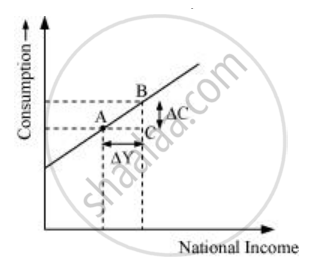Advertisement Remove all ads

# What is Marginal Propensity to Consume? How is It Related to Marginal Propensity to Save? - Economics

Answer in Brief

What is marginal propensity to consume? How is it related to marginal propensity to save?

Advertisement Remove all ads

#### Solution

Marginal propensity to consume refers to the ratio of change in the consumer’s expenditure due to the change in disposable income (income after deducting taxes). In other words, MPC measures how consumption will vary with the change in income.

So,

MPC=(DeltaC)/(DeltaY)

Where,

ΔC = Change in consumption

ΔY = Change in income

For example, if income increases from Rs 200 crores to Rs 250 crores and consumption increases from Rs 20 crores to Rs 40 crores, it implies that 0.4 is the MPC or 40% increase in the income is being consumed.

This can further be explained with the help of a table and a diagram.

If income and consumption are:-

 Income in Rs (Y) Consumption Expenditure in Rs (C) 200 20 250 40

Then MPC=(DeltaC)/(DeltaY)=20/50=0.4Also, MPC can be explained with the given diagram.

In the diagram, x-axis represents national income and y-axis represents consumption level.

So, MPC=(BC)/(AC)

The relationship between MPC and MPS can be explained as

Y = C + S (Assuming that the income earned is either consumed or saved)

Or, ΔY = ΔC + ΔS

Dividing both sides by ΔY

(DeltaY)/(DeltaY)=(DeltaC)/(DeltaY)+(DeltaS)/(DeltaY).............[(DeltaS)/(DeltaY)=MPS]

Or, 1 = MPC + MPS

Or, MPC = 1 − MPS

Or, MPS = 1 − MPC

So, the sum of MPC and MPS is always equal to unity.

Concept: Aggregate Demand and Its Components - Consumption
Is there an error in this question or solution?
Advertisement Remove all ads

#### APPEARS IN

NCERT Class 12 Economics - Introductory Macroeconomics
Chapter 4 Determination of Income And Employment
Exercise | Q 1 | Page 65
Advertisement Remove all ads
Advertisement Remove all ads
Share
Notifications

View all notifications

Forgot password?